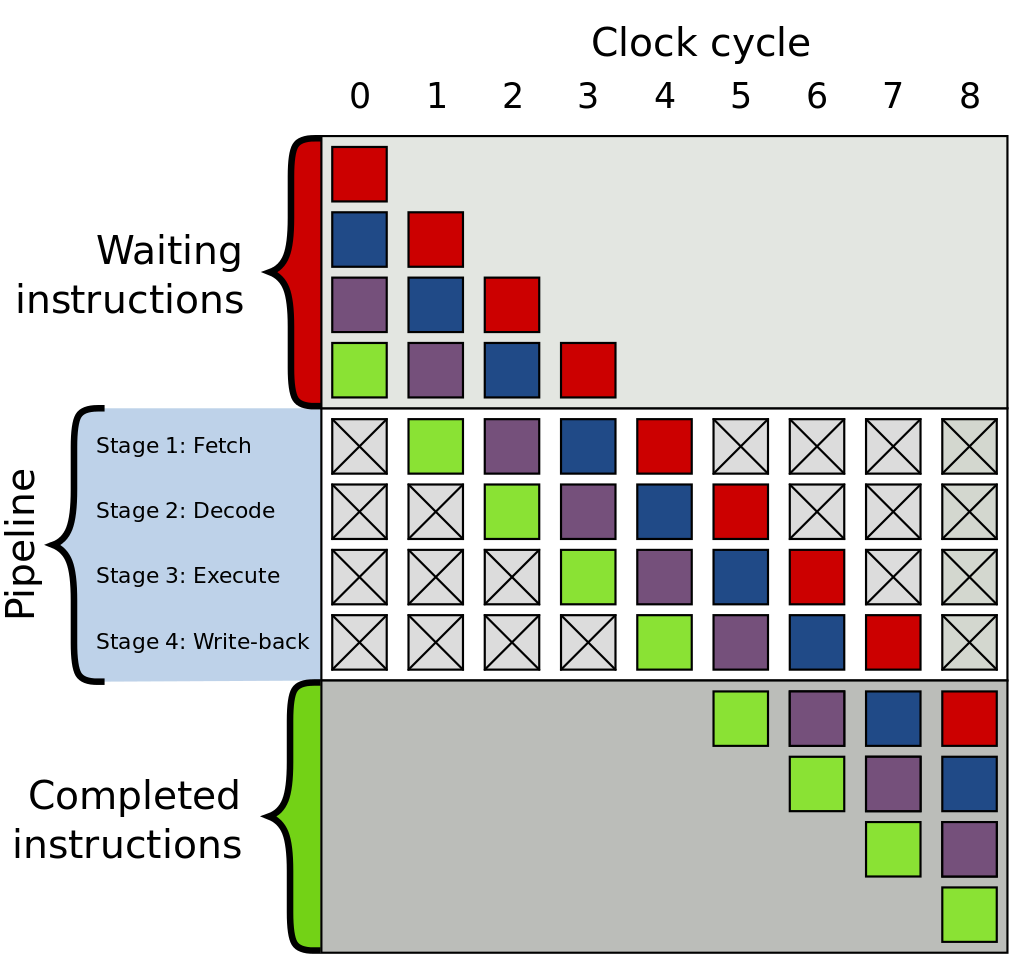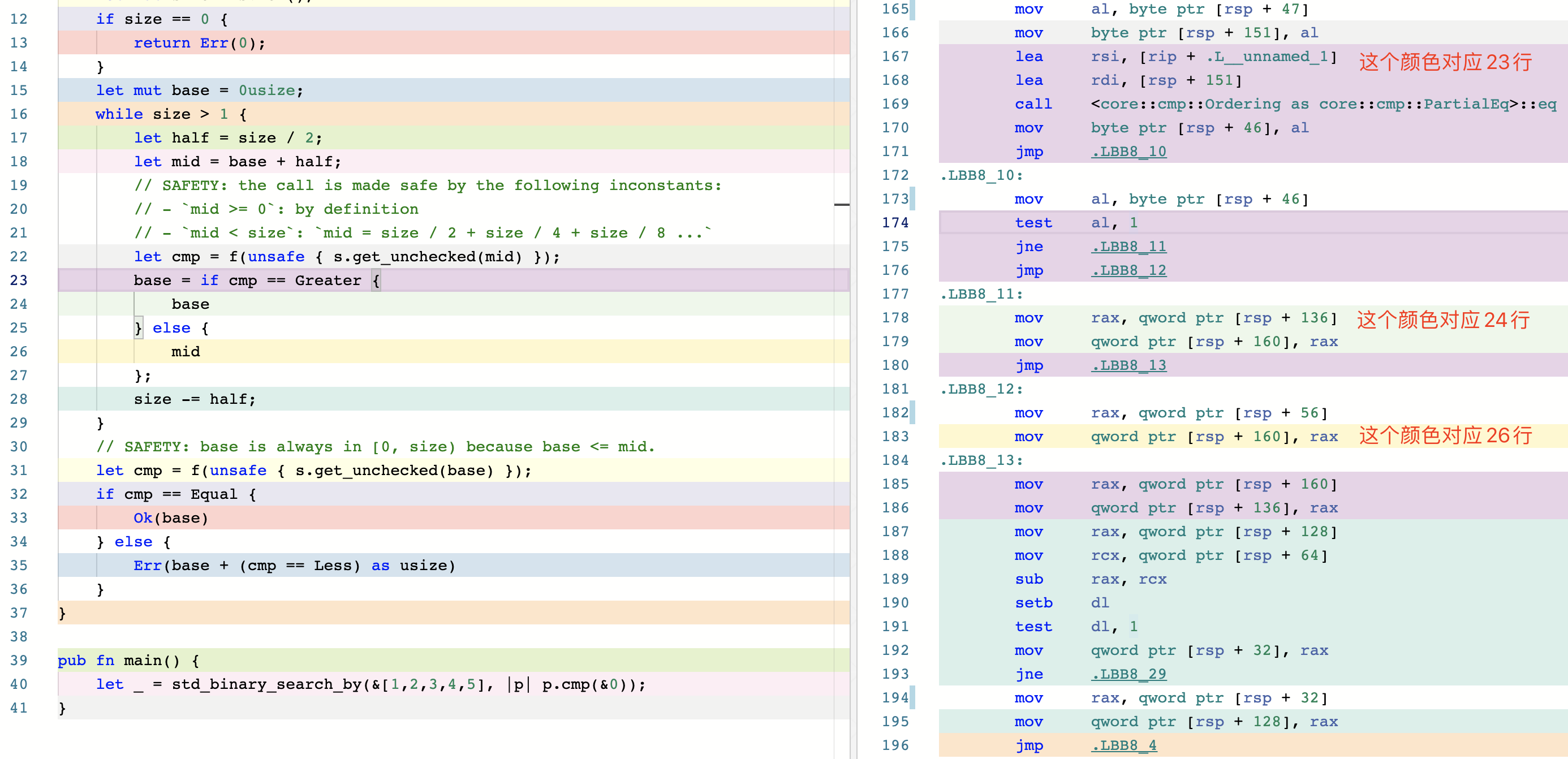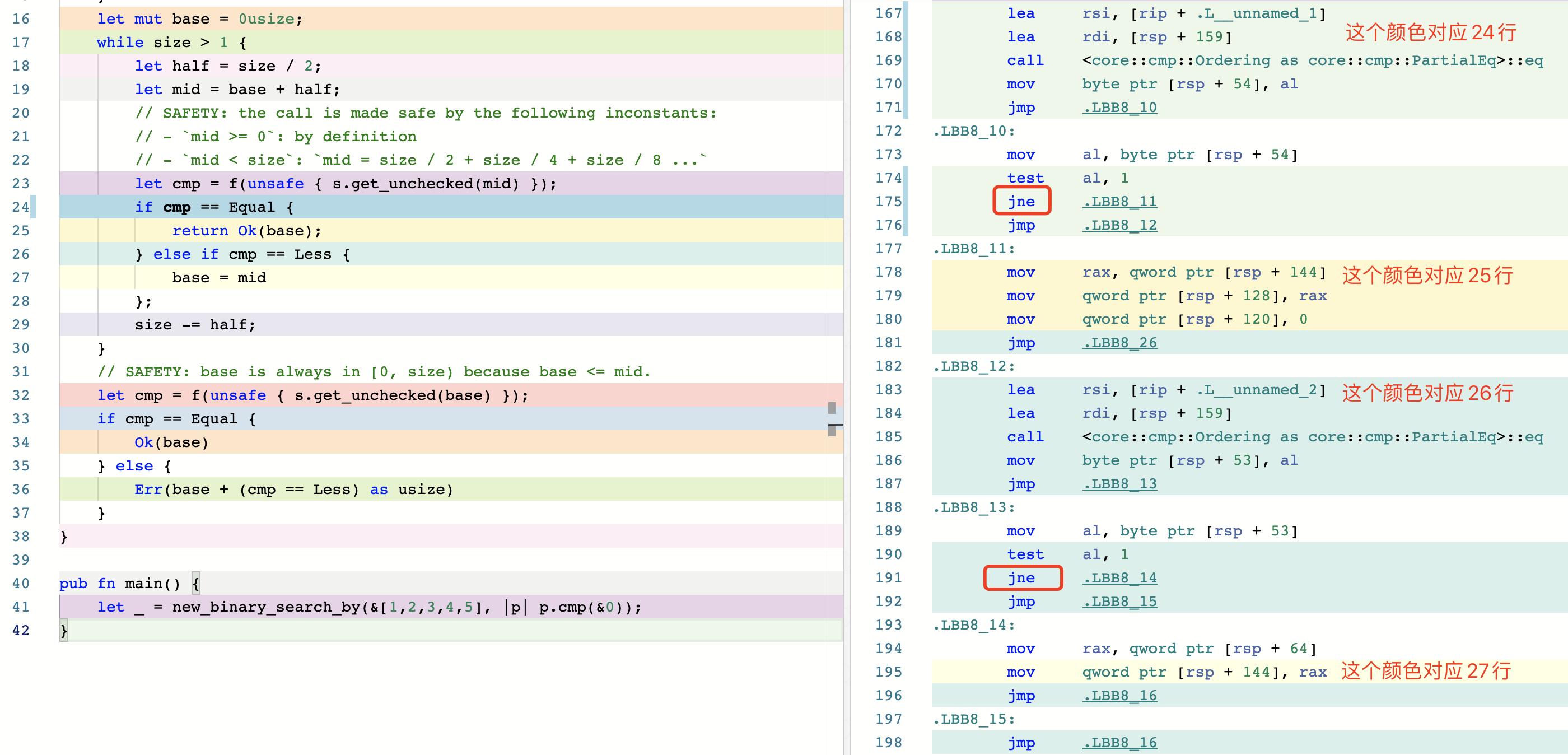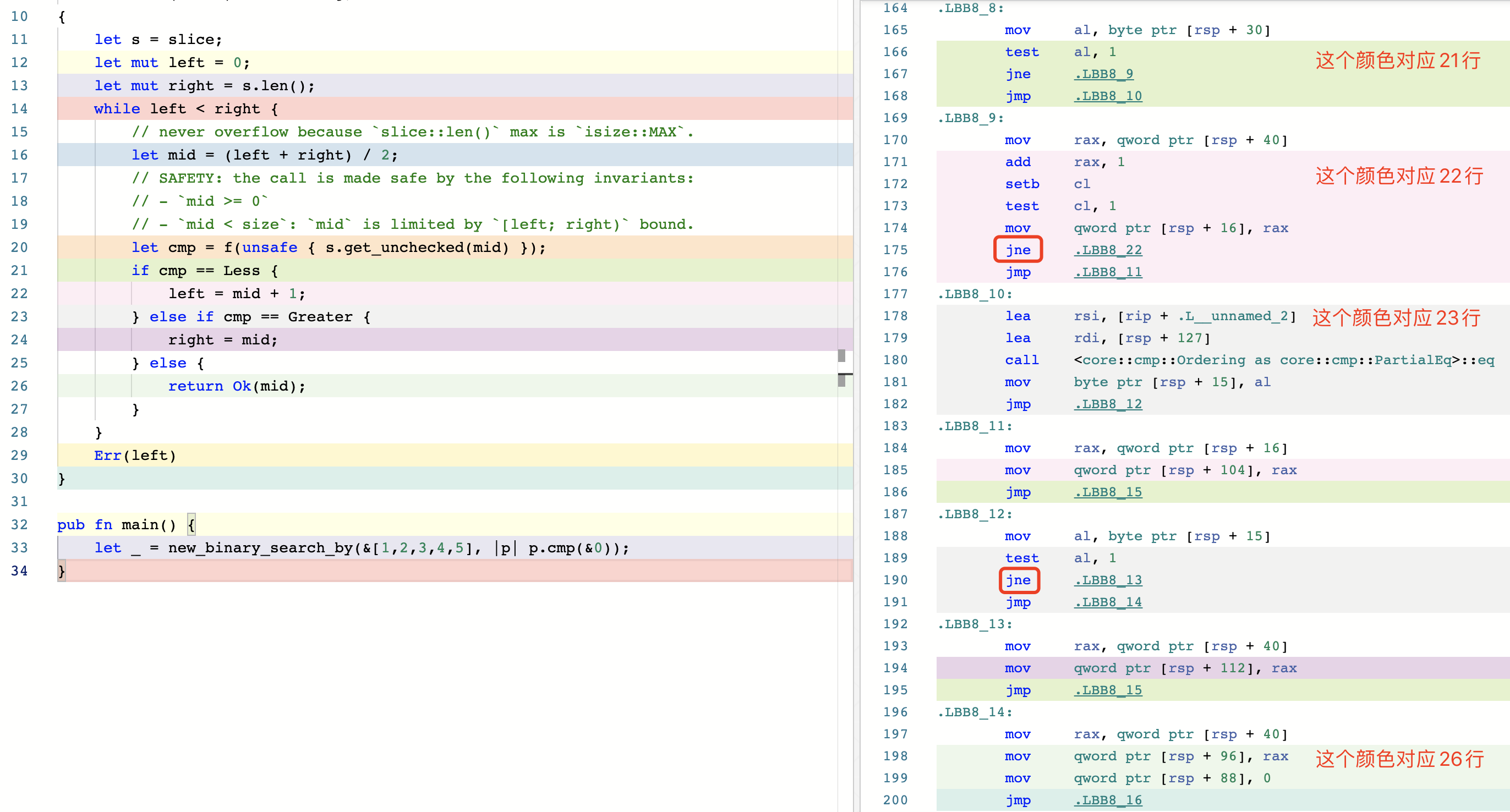# 优化 Rust 标准库的 binary_search

2021 年 5 月 6 号发布的 Rust 1.52 版将会包含我的一个 PR，将标准库 slice::binary_search_by() 的最好时间复杂度优化到 O(1)。PR 编号 #74024，从 2020 年 7 月初提交的 PR，到 2021 年 3 月 6 号才正式合并，其中包含 70 多条评论，前后历时大半年。这个 PR 虽然改动不算很大，但是笔者在其中也学到了不少知识点，所以特意写一篇文章总结一下，希望能帮助到大家。


#![allow(unused)]
fn main() {
let s = [0, 1, 1, 1, 1, 2, 3, 5, 8, 13, 21, 34, 55];

let seek = 13;
assert_eq!(s.binary_search_by(|probe| probe.cmp(&seek)), Ok(9));
let seek = 4;
assert_eq!(s.binary_search_by(|probe| probe.cmp(&seek)), Err(7));
let seek = 100;
assert_eq!(s.binary_search_by(|probe| probe.cmp(&seek)), Err(13));
let seek = 1;
let r = s.binary_search_by(|probe| probe.cmp(&seek));
assert!(match r { Ok(1..=4) => true, _ => false, });
}



#![allow(unused)]
fn main() {
#[stable(feature = "rust1", since = "1.0.0")]
#[inline]
pub fn binary_search_by<'a, F>(&'a self, mut f: F) -> Result<usize, usize>
where
F: FnMut(&'a T) -> Ordering,
{
let s = self;
let mut size = s.len();
if size == 0 {
return Err(0);
}
let mut base = 0usize;
while size > 1 {
let half = size / 2;
let mid = base + half;
// SAFETY: the call is made safe by the following inconstants:
// - mid >= 0: by definition
// - mid < size: mid = size / 2 + size / 4 + size / 8 ...
let cmp = f(unsafe { s.get_unchecked(mid) });
base = if cmp == Greater { base } else { mid };
size -= half;
}
// SAFETY: base is always in [0, size) because base <= mid.
let cmp = f(unsafe { s.get_unchecked(base) });
if cmp == Equal { Ok(base) } else { Err(base + (cmp == Less) as usize) }
}
}



#![allow(unused)]
fn main() {
while size > 1 {
let half = size / 2;
let mid = base + half;
// SAFETY:
// mid is always in [0, size), that means mid is >= 0 and < size.
// mid >= 0: by definition
// mid < size: mid = size / 2 + size / 4 + size / 8 ...
let cmp = f(unsafe { s.get_unchecked(mid) });
if cmp == Equal {
return Ok(base);
} else if cmp == Less {
base = mid
};
size -= half;
}
}


// 标准库的实现
test slice::binary_search_l1           ... bench:          59 ns/iter (+/- 4)
test slice::binary_search_l1_with_dups ... bench:          59 ns/iter (+/- 3)
test slice::binary_search_l2           ... bench:          76 ns/iter (+/- 5)
test slice::binary_search_l2_with_dups ... bench:          77 ns/iter (+/- 17)
test slice::binary_search_l3           ... bench:         183 ns/iter (+/- 23)
test slice::binary_search_l3_with_dups ... bench:         185 ns/iter (+/- 19)

// 新版实现
test slice::binary_search_l1           ... bench:          58 ns/iter (+/- 2)
test slice::binary_search_l1_with_dups ... bench:          37 ns/iter (+/- 4)
test slice::binary_search_l2           ... bench:          76 ns/iter (+/- 3)
test slice::binary_search_l2_with_dups ... bench:          57 ns/iter (+/- 6)
test slice::binary_search_l3           ... bench:         200 ns/iter (+/- 30)
test slice::binary_search_l3_with_dups ... bench:         157 ns/iter (+/- 6)


## 分支预测(Branch prediction)

### 分支

jmpAlways jump
jeJump if cmp is equal
jneJump if cmp is not equal
jgSigned > (greater)
jgeSigned >=
jlSigned < (less than)
jleSigned <=
jaUnsigned > (above)
jaeUnsigned >=
jbUnsigned < (below)
jbeUnsigned <=
jecxzJump if ecx is 0
jcJump if carry: used for unsigned overflow, or multiprecision add
joJump if there was signed overflow

#![allow(unused_assignments)]
pub fn main() {
// 接收用户从命令行中输入的参数
let mut a: usize = std::env::args().nth(1).unwrap().parse().unwrap_or_defaul();
if a > 42 {
a = 1;
} else {
a = 0;
}
}


.LBB99_7:
cmp     qword ptr [rsp + 56], 42 ; if a > 42
jbe     .LBB99_10
mov     qword ptr [rsp + 56], 1  ; a = 1
jmp     .LBB99_11
.LBB99_10:
mov     qword ptr [rsp + 56], 0  ; a = 0
.LBB99_11:
ret


### 指令流水线(instruction pipelining)

CPU 处理一条指令一般要分为几个步骤：

• 取指令(Instruction Fetch)
• 解指令(Instruction Decode)
• 执行指令 (Execute)
• 回写结果到寄存器 (Register write back)

Wikipedia 上的这张图可以帮助理解（图中文字颜色为黑色，推荐用 Light 主题查看）。### 为什么需要分支预测？

CPU 的分支预测器会提前预测这条 jump 指令可能会跳转的到哪个分支，然后把预测的那条分支放到流水线中去执行。如果预测正确，CPU 可以继续执行后面的指令；如果预测失败了(branch misprediction)，那只能丢弃刚刚执行的分支结果，重新切换到正确的分支执行。可以看到，如果出现过多的预测失败，分支预测反而很影响性能。不过现在 CPU 的分支预测器经过这么多年的发展已经越来越先进了，人们会采用各种方式不断提高分支预测器的预测准确率，详细可以查看 Wikipedia 的 Branch_predictor 了解更多。

### 热循环中避免分支预测


#![allow(unused)]
fn main() {
pub struct Filter<I, P> {
iter: I,
predicate: P,
}

impl<I: Iterator, P> Iterator for Filter<I, P>
where
P: FnMut(&I::Item) -> bool,
{
type Item = I::Item;

// this special case allows the compiler to make .filter(_).count()
// branchless. Barring perfect branch prediction (which is unattainable in
// the general case), this will be much faster in >90% of cases (containing
// virtually all real workloads) and only a tiny bit slower in the rest.
//
// Having this specialization thus allows us to write .filter(p).count()
// where we would otherwise write .map(|x| p(x) as usize).sum(), which is
// less readable and also less backwards-compatible to Rust before 1.10.
//
// Using the branchless version will also simplify the LLVM byte code, thus
// leaving more budget for LLVM optimizations.
#[inline]
fn count(self) -> usize {
#[inline]
fn to_usize<T>(mut predicate: impl FnMut(&T) -> bool) -> impl FnMut(T) -> usize {
move |x| predicate(&x) as usize
}

self.iter.map(to_usize(self.predicate)).sum()
}
}
}



#![allow(unused)]
fn main() {
#[inline]
fn count(self) -> usize {
let sum = 0;

self.iter.for_each(|x| {
if self.predicate(x) {
sum += 1;
}
});

sum
}
}


• 关于怎样写好 branchless code 来优化性能又是另一个值得专门讨论的主题了，网上也有大量资料可以学习。但是 branchless code 会牺牲不少代码可读性，并不建议盲目的使用。

• branchless code 的额外好处是还能帮助避免遭受旁路攻击(英文 timing attack 或者 side-channel attack)。参考：https://en.wikipedia.org/wiki/Timing_attack

• 标准库的汇编截图• 优化(1)的汇编截图## 优化（2）


#![allow(unused)]
fn main() {
pub fn binary_search_by<'a, F>(&'a self, mut f: F) -> Result<usize, usize>
where
F: FnMut(&'a T) -> Ordering,
{
let mut left = 0;
let mut right = self.len();
while left < right {
// never overflow because slice::len() max is isize::MAX.
let mid = (left + right) / 2;
// SAFETY: the call is made safe by the following invariants:
// - mid >= 0
// - mid < size: mid is limited by [left; right) bound.
let cmp = f(unsafe { self.get_unchecked(mid) });
if cmp == Less {
left = mid + 1;
} else if cmp == Greater {
right = mid;
} else {
return Ok(mid);
}
}
Err(left)
}
}crater 大概就是针对整个 crates.io 上所有的 crate 给要测试的编译器版本（比如我的 PR ）跑一次测试，看这个改动对线上所有 crate 影响大不大。crates.io 上超过5万个 crate，一般跑一次 crater 需要将近一周的时间。我的这个 crater 跑完之后就是因为没有对已发布的 crate 造成什么很大的影响，所以官方才愿意合并。

From m-ou-se:

"We discussed this PR in a recent library team meeting, in which we agreed that the proposed behaviour (stopping on Equal) is preferrable over optimal efficiency in some specific niche cases. Especially considering how small most of the differences are in the benchmarks above."

"The breakage in the crater report looks reasonably small. Also, now that partition_point is getting stabilized, there's a good alternative for those who want the old behaviour of binary_search_by. So we should go ahead and start on getting this merged. :)"

## 整数溢出问题


#![allow(unused)]
fn main() {
// never overflow because slice::len() max is isize::MAX.
let mid = (left + right) / 2;
}


slice::len() 的返回值是 usize 类型，但是对于非零大小的类型(non-ZST)，slice::len() 的值最大也只能是 isize::MAX。所以就像注释上写的那样 (isize::MAX + isize::MAX) / 2 是不能可能超过 usize::MAX 的，所以不会发生 overflow。但是对于 ZST 类型就不一样了，如果 slice 里面所有元素都是零大小的（比如 ()），那这个 slice 的长度完全可以达到 usize::MAX。虽然对于 [(); usize::MAX].binary_search(&()) 这种情况我们会在 O(1) 的时间复杂度上找到结果并马上返回，但是如果我们这么写 b.binary_search_by(|_| Ordering::Less)，它就发生整数溢出了。

### 为什么 slice::len() 对于 non-ZST 最大值是 isize 呢？

fn main() {
assert_eq!(std::mem::size_of::<bool>(), 1);
// error: values of the type [bool; 18446744073709551615] are too big
// for the current architecture
let _s = [true; usize::MAX];
}


fn main() {
assert_eq!(std::mem::size_of::<()>(), 0);
let s = [(); usize::MAX];
assert_eq!(s.len(), usize::MAX);
}


## 最终版本


#![allow(unused)]
fn main() {
pub fn binary_search_by<'a, F>(&'a self, mut f: F) -> Result<usize, usize>
where
F: FnMut(&'a T) -> Ordering,
{
let mut size = self.len();
let mut left = 0;
let mut right = size;
while left < right {
let mid = left + size / 2;

// SAFETY: the call is made safe by the following invariants:
// - mid >= 0
// - mid < size: mid is limited by [left; right) bound.
let cmp = f(unsafe { self.get_unchecked(mid) });
// The reason why we use if/else control flow rather than match
// is because match reorders comparison operations, which is
// perf sensitive.
// This is x86 asm for u8: https://rust.godbolt.org/z/8Y8Pra.
if cmp == Less {
left = mid + 1;
} else if cmp == Greater {
right = mid;
} else {
return Ok(mid);
}

size = right - left;
}
Err(left)
}

#[test]
fn test_binary_search_by_overflow() {
let b = [(); usize::MAX];
assert_eq!(b.binary_search_by(|_| Ordering::Equal), Ok(usize::MAX / 2));
assert_eq!(b.binary_search_by(|_| Ordering::Greater), Err(0));
assert_eq!(b.binary_search_by(|_| Ordering::Less), Err(usize::MAX));
}
}


## 关于我

Id: Folyd，GitHub：@folyd。字节跳动飞书 Rust 工程师，Rust Search Extension 作者。